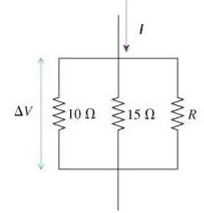# What is the value of resistor R in the figure if ΔV=8V and I=6A?

What is the value of resistor R in the figure if ΔV=8V and I=6A?
figure